# Incorrect LaTeX export of "norm" delimiters

I am cross posting a converter bug I found. Texmacs does not export resized norm symbols correctly. Instead, it often adds arbitrary bars mangling the math.

Ignored resizing

Consider the difference between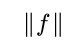and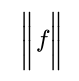in which the norm symbol has been made larger. The change gets ignored by the exporter that produces identical output:

$\| f \|$


Now consider a case where resizing is actually appropriate e.g.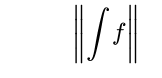(this is the normal size that TeXmacs decides on)
vs an “enlarged” version: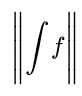or a “reduced” version:The former exports correctly to
$\left\| \int f \right\|$
while the latter two export to gibberish
$\left\|| \int f \right\||$
This gibberish adds extra bars in LaTeX. Here is sample PDF outfut from LaTeX.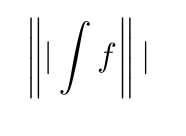If there are extra things in the formula the result, imaginably, is a completely mangled equation.

This makes LaTeX export very inconvenient. One has to literally go through the whole paper by hand and correct all norms.

Here is the bug report: GNU TeXmacs - Bugs: bug #62828, Incorrect LaTeX export of… [Savannah]

2 Likes

Meanwhile, the following sed script takes care of the issue:

s#$$[ {]\\\(b\|B$$ig*$$l\|r$$\?\\|\)|$$[ _}^]$$#\1\4#g
s#$$[ {]\\left\\|$$|$$[ _}^]$$#\1\2#g
s#$$[ {]\\right\\|$$|$$[ _}^]$$#\1\2#g


Save that to a file e.g. norm-bug.sed
and run
sed -e norm-bug.sed filename.tex > filename.out.tex
and your shining tex file will be in filename.out.tex. If you trust the script you can also run

sed -i -e norm-bug.sed filename.tex

that will modify the tex file in place.

Note: I add the checking for a space or a { and for one of the characters _}^ after the culprit because I to avoid false positives. Maybe one could remove it all and simply have

s#$$\\\(b\|B$$ig*$$l\|r$$\?\\|\)|#\1#g
s#$$\\left\\|$$|#\1#g
s#$$\\right\\|$$|#\1#g


If you are having trouble, let me know and I can debug a better workaround for now.

EDIT: changed the sed script for a corner case.

2 Likes